library(bbmle)
## Loading required package: stats4
library(emdbook)
library("ggplot2"); theme_set(theme_bw())

## Data

# Initial: microalgae cells
# Killed: consumed units by cladoceran filter feeder
d <- data.frame(Initial=c(100000,100000,100000,
100000,500000,500000,500000,500000,2000000,
2000000,2000000,2000000,4000000,4000000,4000000,
6000000,6000000,6000000,6000000),
Killed=c(3750,5625,3125,3125,13125,16250,15625,12500,
37500,31250,40625,28125,53125,62500,46875,62500,
71875,59375,50000))

Initial notes:

• On a purely numerical note, we might be in trouble because of scaling issues unless we go carefully.
• On a statistical note, with such huge numbers a binomial is not actually likely to be appropriate – the expected sampling variation will be very small, so we’re likely to get overly small numbers. Also, are these actual counts, or are they “units” consumed, i.e. not identifiable with discrete individuals? If the latter, then we have to give up on binomial fits anyway.

Programming notes:

• using attach() is almost always a bad idea
• using data as a variable is less bad, but also should be avoided (it occasionally gives odd/surprising errors)
(gg0 <- qplot(Initial,Killed,data=d))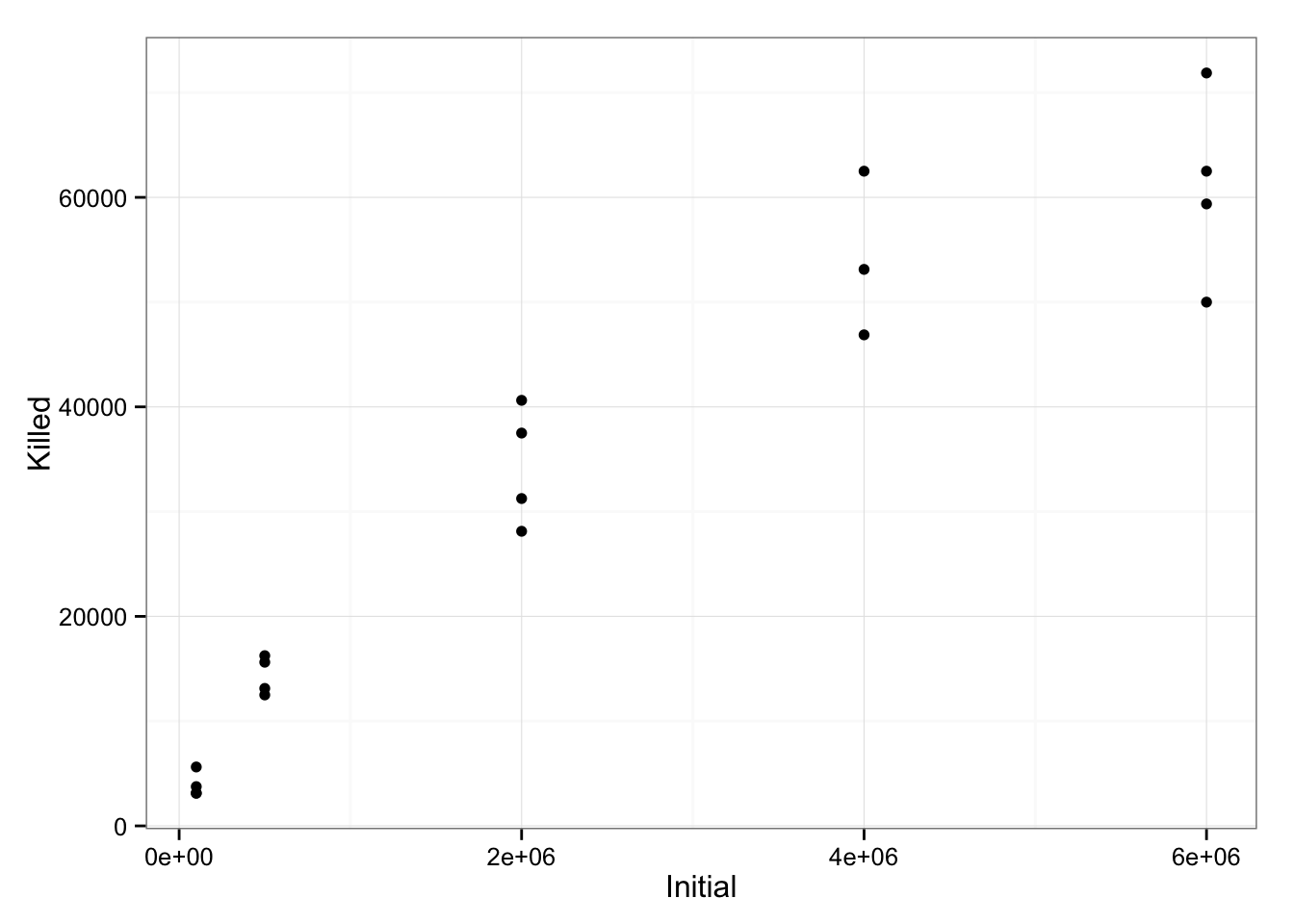Initial estimates (Holling = $$aN/(1+ahN)$$: asymptote is around $$10^5$$, so $$h \approx 10^{-5}$$; initial slope ($$a$$) is around 0.01. A bit of playing around gives $$a=0.025$$ as a better Holling fit.

h2 <- function(x,a=0.025,h=1e-5) a*x/(1+a*h*x)
gg0 + stat_function(fun=h2)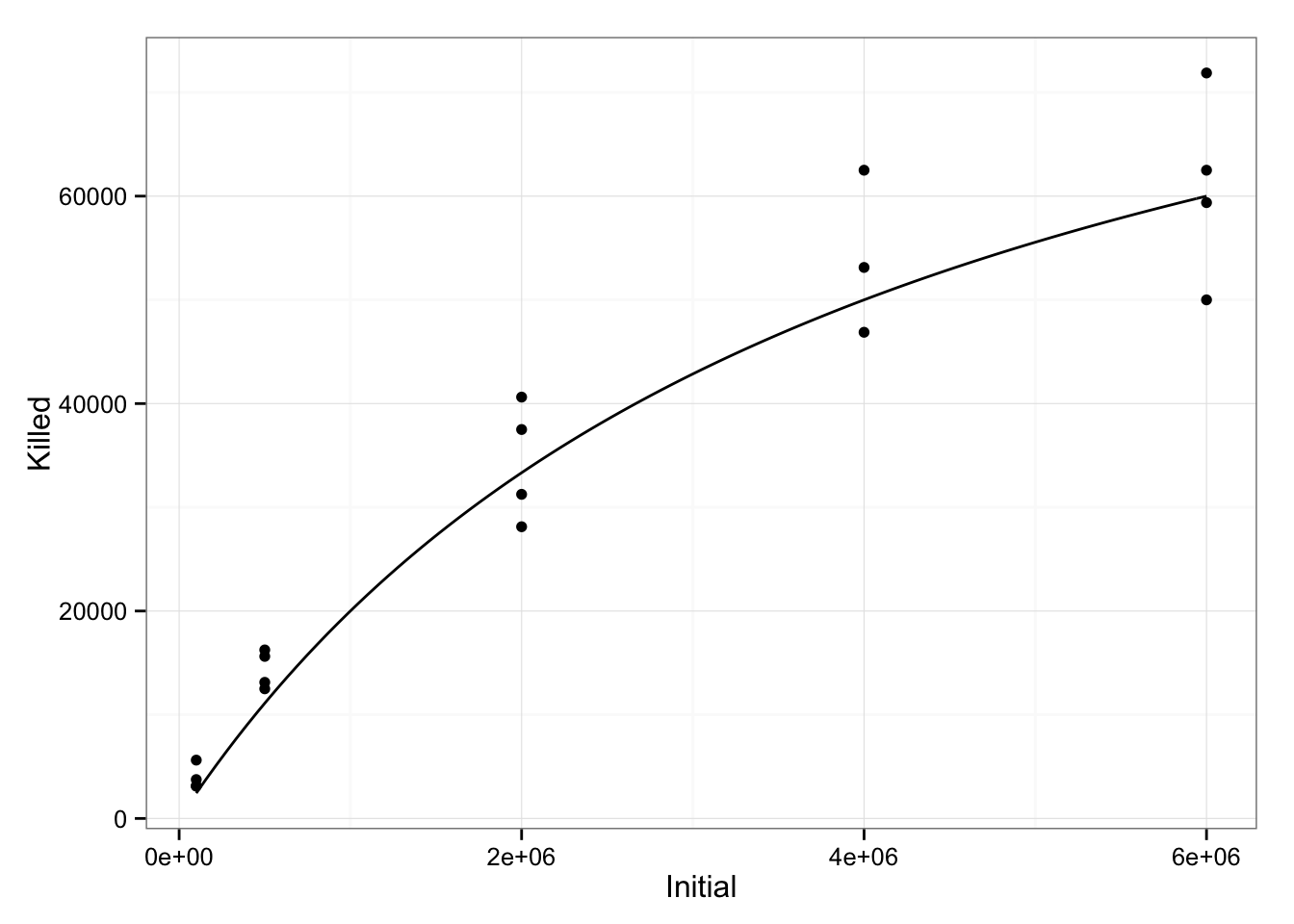## Rogers equation

(Note it’s “Rogers”, not “Roger’s” …)

rogers.pred <- function(N0,a,h)  {
N0 - lambertW(a*h*N0*exp(-a*(1-h*N0)))/(a*h)
}
NLL.rogers <- function(a, h, Initial, Killed) {
if (a < 0 || h < 0)
return(NA)
prop.exp = rogers.pred(Initial,a,h)/Initial
-sum(dbinom(Killed, prob = prop.exp, size = Initial,log = TRUE))
}

### Fit

Let’s see if we can do a nonlinear least-squares fit first.

(n1 <- nls(Killed~rogers.pred(Initial,a,h),
start=list(a=0.025,h=1e-5),
data=d))
## Nonlinear regression model
##   model: Killed ~ rogers.pred(Initial, a, h)
##    data: d
##         a         h
## 3.008e-02 1.074e-05
##  residual sum-of-squares: 520066688
##
## Number of iterations to convergence: 4
## Achieved convergence tolerance: 3.614e-07

This seems to work OK.

Let’s go ahead and do a least-squares fit for Holling type II so we can compare.

(n0 <- nls(Killed~a*Initial/(1+a*h*Initial),
start=list(a=0.025,h=1e-5),
data=d))
## Nonlinear regression model
##   model: Killed ~ a * Initial/(1 + a * h * Initial)
##    data: d
##         a         h
## 0.0297356 0.0000107
##  residual sum-of-squares: 519815132
##
## Number of iterations to convergence: 4
## Achieved convergence tolerance: 3.999e-07
(gg0B <- gg0 + stat_function(fun=function(x) h2(x,a=coef(n0)["a"],h=coef(n0)["h"]))+
stat_function(fun=function(x,a=coef(n1)["a"],h=coef(n1)["h"]) {
rogers.pred(x,a,h)
},colour="red",linetype=2))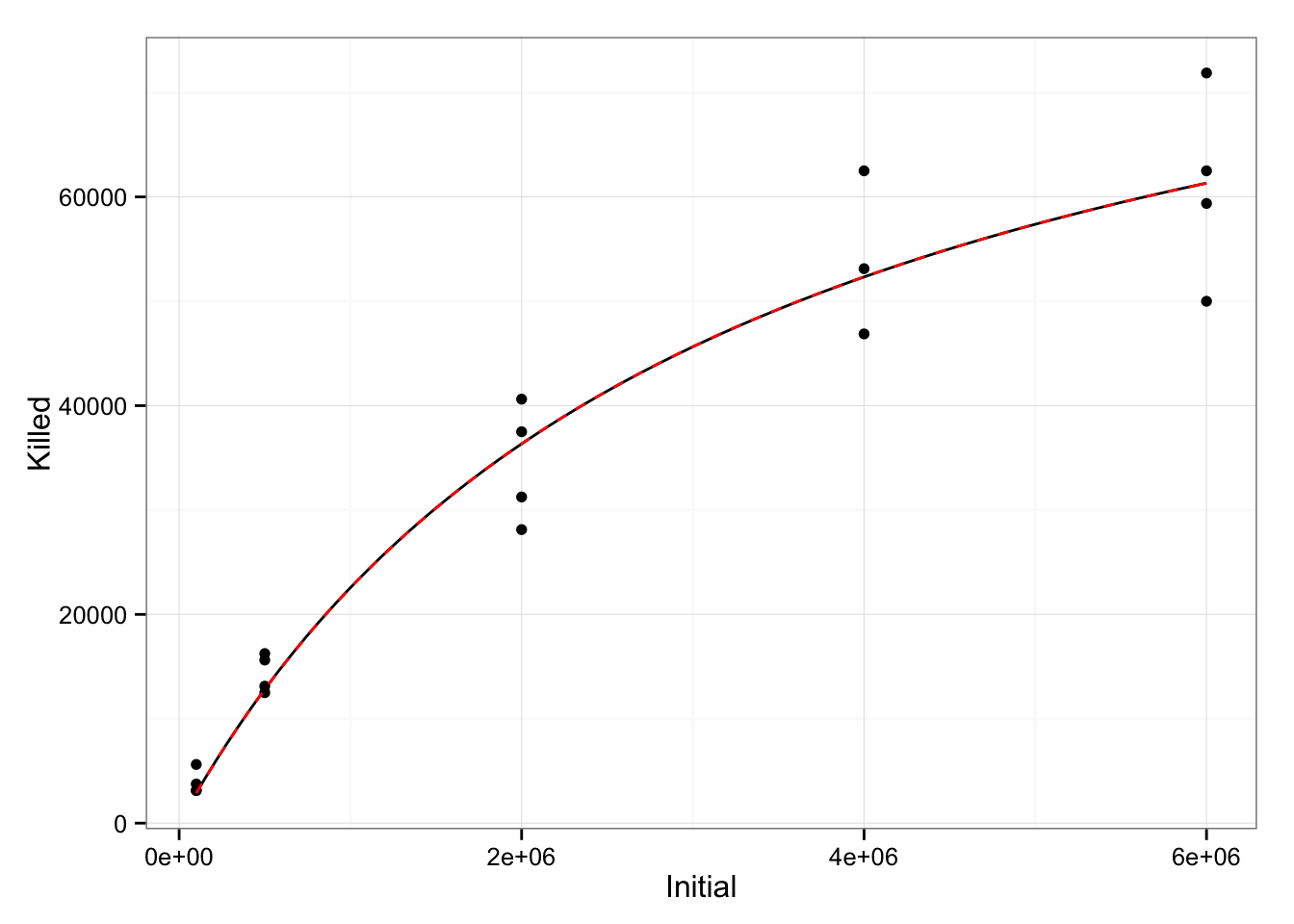The fits are nearly indistinguishable (using linetype=2 makes it easier to see that both lines are actually there).

Put together the parameters and the confidence intervals and compare:

cc0 <- confint(n0)
## Waiting for profiling to be done...
cc1 <- confint(n1)
## Waiting for profiling to be done...
partab <- cbind(var=rep(c("a","h"),2),
rbind(data.frame(m="holling",cbind(coef(n0),cc0)),
data.frame(m="rogers",cbind(coef(n1),cc1))))
names(partab)[3:5] <- c("est","lwr","upr")
(gg1 <- ggplot(partab,aes(var,est,colour=m))+
geom_pointrange(aes(ymin=lwr,ymax=upr),
position=position_dodge(width=0.1))+
facet_wrap(~var,scale="free"))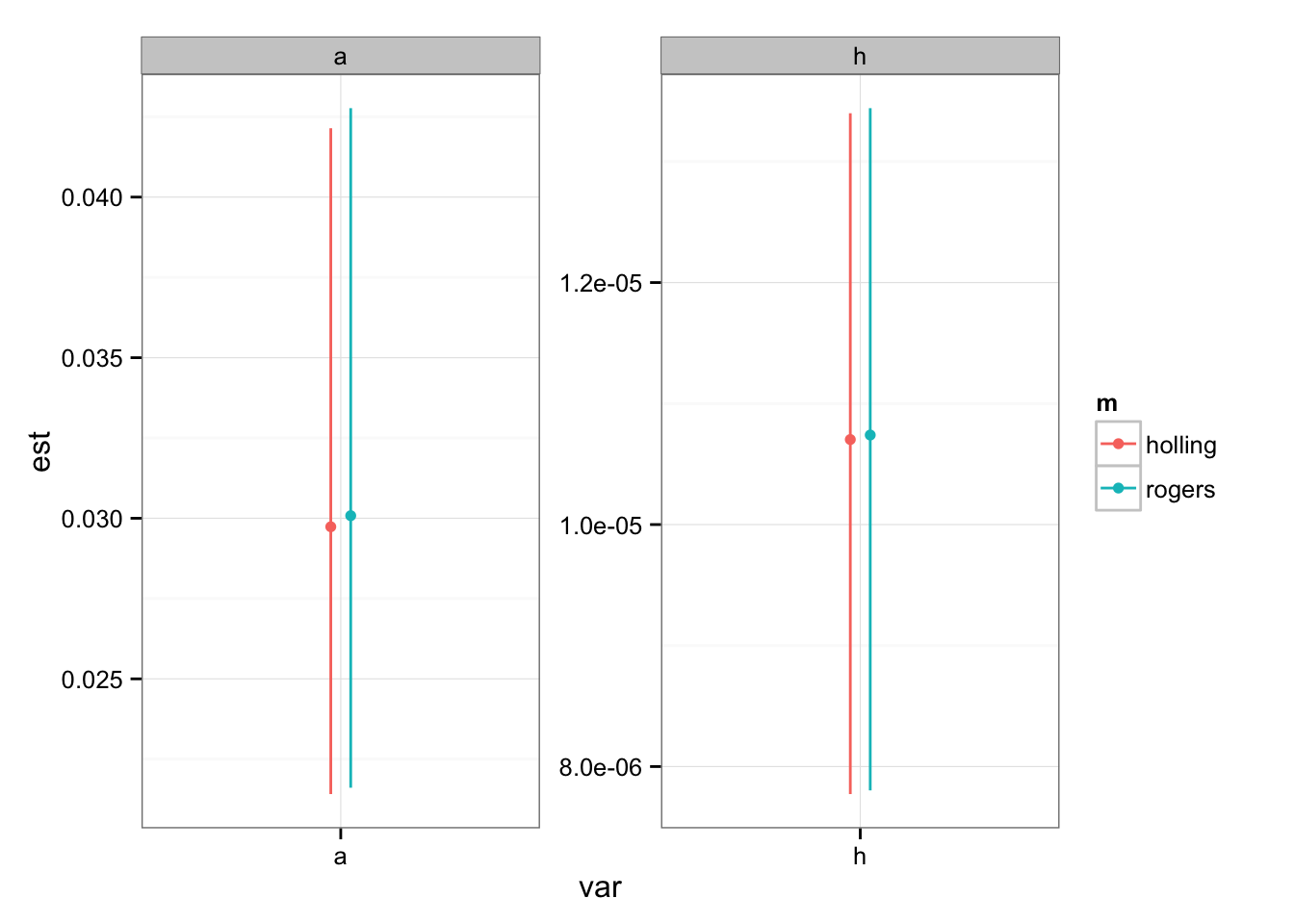Now we can fit by binomial MLE, for comparison:

## the key here is parscale=coef(n1) [as well as starting with
## good parameter values]
FFR.rogers <- mle2(NLL.rogers, start = list(a=coef(n1)["a"],h=coef(n1)["h"]),
data=d,control=list(parscale=coef(n1)))
(n2 <- coef(FFR.rogers))
##            a            h
## 3.368721e-02 1.162603e-05
(cc2 <- confint(FFR.rogers))
##          2.5 %       97.5 %
## a 3.340906e-02 3.396527e-02
## h 1.154535e-05 1.170552e-05
partab2 <- rbind(partab,
data.frame(var=c("a","h"),
m="rogers_binom",
est=coef(FFR.rogers),
lwr=cc2[,1],upr=cc2[,2]))
gg1 %+% partab2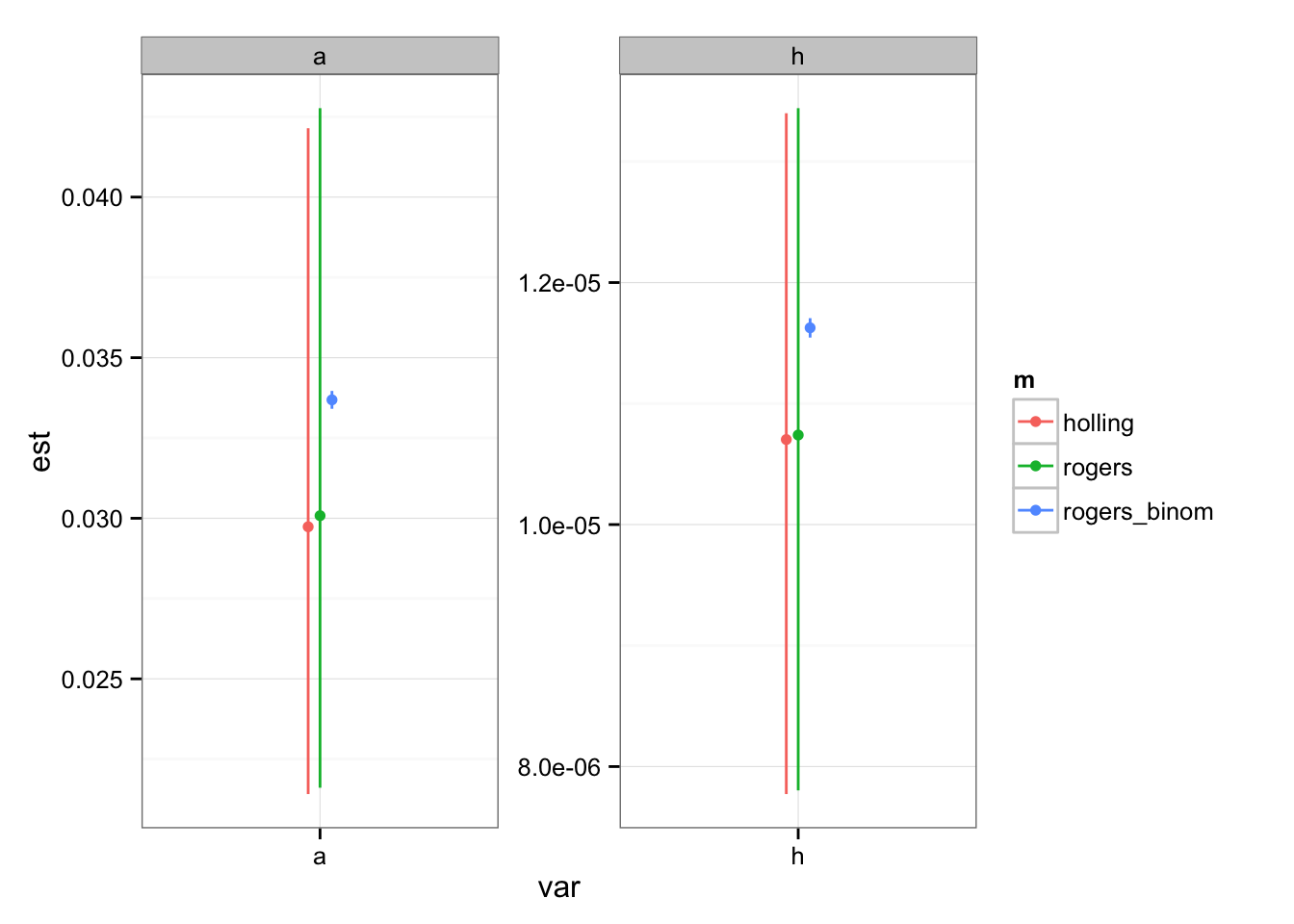The binomial fit is somewhat different from the NLS fit, but not really biologically significantly different …

(gg0C <- gg0B + stat_function(fun=function(x,a=n2["a"],h=n2["h"]) {
rogers.pred(x,a,h)
},colour="blue"))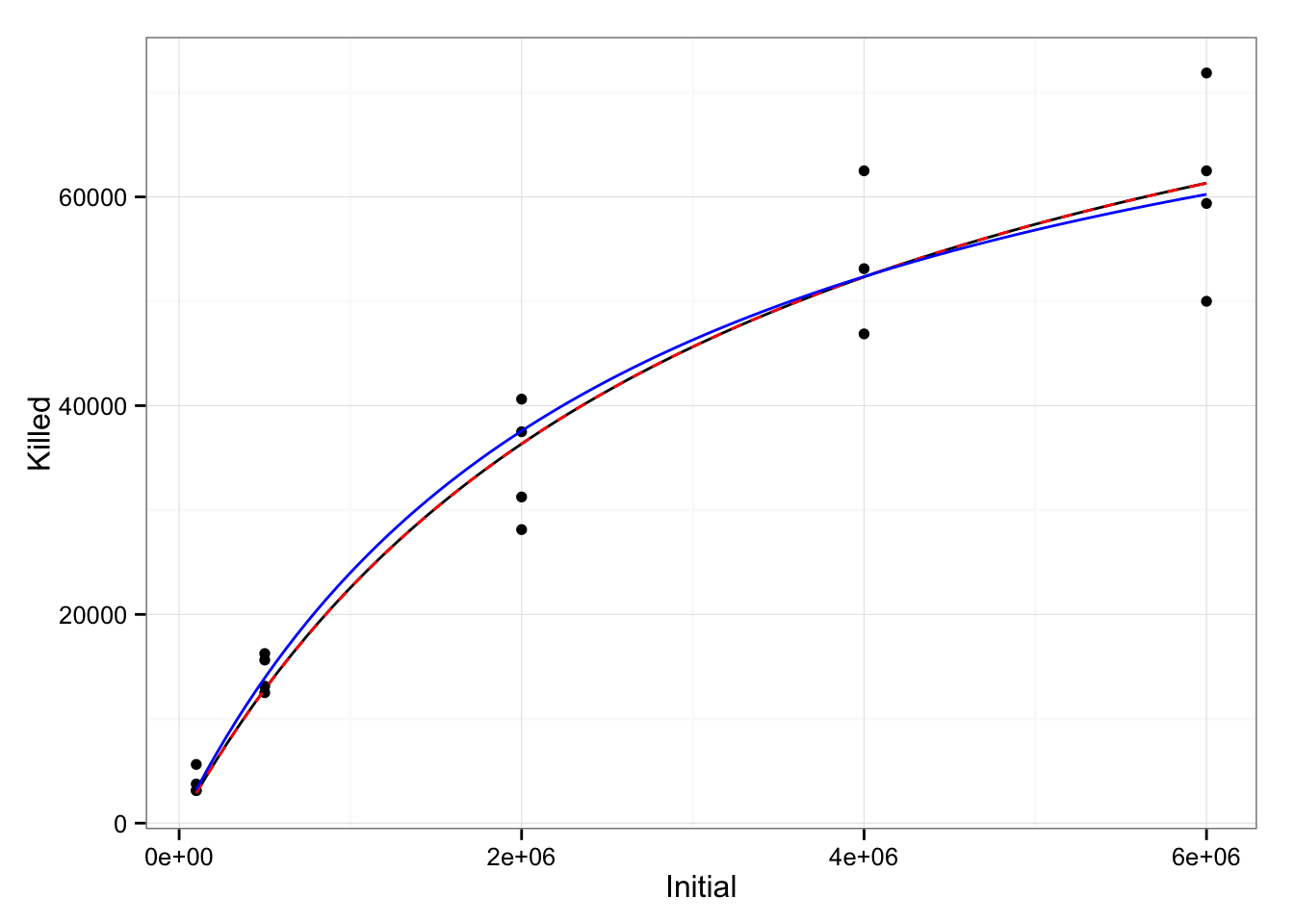The confidence intervals on the binomial are most likely severely understated. A quick estimate of the overdispersion is

(od <- deviance(FFR.rogers)/(nrow(d)-2))
## 'log Lik.' 783.6263 (df=2)

which should be $$\approx 1$$; crudely speaking, we should be multiplying the confidence interval width by about $$\sqrt{\phi} \approx 28$$ (a “quasi-likelihood” approach), or re-fitting the model with a beta-binomial likelihood (dbetabinom() from the emdbook package) or a logit-normal-binomial likelihood (harder).

The bottom line, though, is that for this problem the NLS fit is probably just fine – and in fact even a Holling type II NLS fit is fine.